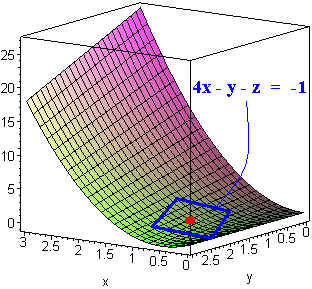$$\newcommand{\id}{\mathrm{id}}$$ $$\newcommand{\Span}{\mathrm{span}}$$ $$\newcommand{\kernel}{\mathrm{null}\,}$$ $$\newcommand{\range}{\mathrm{range}\,}$$ $$\newcommand{\RealPart}{\mathrm{Re}}$$ $$\newcommand{\ImaginaryPart}{\mathrm{Im}}$$ $$\newcommand{\Argument}{\mathrm{Arg}}$$ $$\newcommand{\norm}{\| #1 \|}$$ $$\newcommand{\inner}{\langle #1, #2 \rangle}$$ $$\newcommand{\Span}{\mathrm{span}}$$

# 1.7: Tangent Planes and Normal Lines

$$\newcommand{\vecs}{\overset { \rightharpoonup} {\mathbf{#1}} }$$

$$\newcommand{\vecd}{\overset{-\!-\!\rightharpoonup}{\vphantom{a}\smash {#1}}}$$

## Tangent Planes

Let $$z = f(x,y)$$ be a function of two variables. We can define a new function $$F(x,y,z)$$ of three variables by subtracting $$z$$. This has the condition

$F(x,y,z) = 0.$

Now consider any curve defined parametrically by

$x = x(t), \;\;\; y = y(t), \;\;\; z = z(t).$

We can write,

$F(x(t), y(t), z(t)) = 0.$

Differentiating both sides with respect to $$t$$, and using the chain rule gives

$F_x(x, y, z) x' + F_y(x, y, z) y' + F_z(x, y, z) z' = 0$

Notice that this is the dot product of the gradient function and the vector $$\langle x',y',z'\rangle$$,

$\nabla F \cdot \langle x', y', z'\rangle = 0.$

In particular the gradient vector is orthogonal to the tangent line of any curve on the surface. This leads to:

Definition: Tangent Plane

Let $$F(x,y,z)$$ define a surface that is differentiable at a point $$(x_0,y_0,z_0)$$, then the tangent plane to $$F ( x, y, z )$$ at $$( x_0, y_0, z_0)$$ is the plane with normal vector

$\nabla \, F(x_0,y_0,z_0)$

that passes through the point $$(x_0,y_0,z_0)$$ . In particular, the equation of the tangent plane is

$\nabla \, F(x_0,y_0,z_0) \cdot \langle x - x_0 , y - y_0 , z - z_0 \rangle = 0.$

Example $$\PageIndex{1}$$

Find the equation of the tangent plane to

$z = 3x^2 - xy$

at the point $$(1,2,1)$$.

Solution

We let

$F(x,y,z) = 3x^2 - xy - z$

then

$\nabla F = \langle 6x - y, -x, -1\rangle .$

At the point $$(1,2,1)$$, the normal vector is

$\nabla F(1,2,1) = \langle 4, -1, -1\rangle .$

Now use the point normal formula for a plan

$\langle 4, -1, -1\rangle \cdot \langle x - 1, y - 2, z - 1\rangle = 0$

or

$4(x - 1) - (y - 2) - (z - 1) = 0.$

Finally we get

$4x - y - z = 1.$## Normal Lines

Given a vector and a point, there is a unique line parallel to that vector that passes through the point. In the context of surfaces, we have the gradient vector of the surface at a given point. This leads to the following definition.

Definition: Normal Line

Let $$F(x,y,z)$$ define a surface that is differentiable at a point $$(x_0,y_0,z_0)$$, then the normal line to $$F(x,y,z)$$ at $$(x_0,y_0,z_0)$$ is the line with normal vector

$\nabla \, F(x_0,y_0,z_0) .$

that passes through the point $$(x_0,y_0,z_0)$$ . In Particular the equation of the normal line is

$x(t) = x_0 + F_x(x_0,y_0,z_0) t,$

$y(t) = y_0 + F_y(x_0,y_0,z_0) t,$

$z(t) = z_0 + F_z(x_0,y_0,z_0) t.$

Example $$\PageIndex{2}$$

Find the parametric equations for the normal line to

$x^2yz - y + z - 7 = 0$

at the point $$(1,2,3)$$.

Solution

$\nabla F = \langle 2xyz, x^2z - 1, x^2y + 1\rangle = \langle 12, 2, 3\rangle .$

Now use the formula to find

$x(t) = 1 + 12t, \;\;\; y(t) = 2 + 2t, \;\;\; z(t) = 3 + 3t.$

The diagram below displays the surface and the normal line.## Angle of Inclination

Given a plane with normal vector n the angle of inclination, $$q$$ is defined by

$\cos q = \dfrac{|\textbf{n} \cdot k|}{ ||\textbf{n} ||}.$

More generally, if $$F(x,y,z) = 0$$ is a surface, then the angle of inclination at the point $$(x_0,y_0,z_0)$$ is defined by the angle of inclination of the tangent plane at the point with

$\cos\,q = \dfrac{ | \nabla F(x_0, y_0, z_0) \cdot \textbf{k}| }{|| \nabla F(x_0, y_0, z_0)||}.$

Example $$\PageIndex{3}$$

Find the angle of inclination of

$\dfrac{x^2}{4} + \dfrac{y^2}{4} + \dfrac{z^2}{8} = 1$

at the point $$(1,1,2)$$.

Solution

First compute

$\nabla F = \langle \dfrac{x}{2}, \dfrac{y}{2}, \dfrac{z}{4}\rangle .$

Now plug in to get

$\nabla F(1,1,2) = \langle \dfrac{1}{2}, \dfrac{1}{2}, \dfrac{1}{2} \rangle .$

We have

$|\langle \dfrac{1}{2} , \dfrac{1}{2} , \dfrac{1}{2} \rangle \cdot \hat{\textbf{k}} | = \dfrac{1}{2} .$

Also,

$||\langle \dfrac{1}{2} , \dfrac{1}{2} , \dfrac{1}{2} \rangle || = \dfrac{\sqrt{3}}{2} .$

Hence

$\cos q = \dfrac{\frac{1}{2}}{( \frac{\sqrt{3}}{2} )} = \dfrac{1}{\sqrt{3}} .$

So the angle of inclination is

$q = \cos^{-1}(\dfrac{1}{\sqrt{3}}) = 0.955 \text{ radians} .$

## The Tangent Line to a Curve

Example $$\PageIndex{4}$$

Find the tangent line to the curve of intersection of the sphere

$x^2 + y^2 + z^2 = 30$

and the paraboloid

$z = x^2 + y^2$

at the point $$(1,2,5)$$.

Solution

We find the gradient of the two surfaces at the point

$\nabla(x^2 + y^2 + z^2) = \langle 2x, 2y, 2z\rangle = \langle 2, 4,10\rangle$

and

$\nabla (x^2 + y^2 - z) = \langle 2x, 2y, -1\rangle = \langle 2, 4, -1\rangle .$

These two vectors will both be perpendicular to the tangent line to the curve at the point, hence their cross product will be parallel to this tangent line. We compute

$\begin{vmatrix} \hat{\textbf{i}} & \hat{\textbf{j}} & \hat{\textbf{k}} \\ 2 & 4 & 10 \\ 2 & 4 & -1 \end{vmatrix} = -44 \hat{\textbf{i}} + 22 \hat{\textbf{j}}.$

Hence the equation of the tangent line is

$x(t) = 1 - 44t y(t) = 2 + 22t z(t) = 5.$

Larry Green (Lake Tahoe Community College)

• Integrated by Justin Marshall.